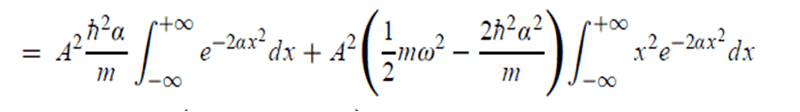# Question in variational method (QM)

## Homework Statement

Hi, in this book " [Nouredine_Zettili]_Quantum_Mechanics_Concepts ", Eq. (9.133)

## Homework Equations

I don't know how the second linehad come from the first line:## The Attempt at a Solution

I got only two terms such that:

$$< \psi_0| H | \psi_0 > = A^2 \int^{+\infty}_{-\infty} \Big( - \frac{2\alpha^2 h^2}{m} e^{-2\alpha x^2} + \frac{1}{2} m \omega^2 x^2 e^{-2\alpha x^2} \Big) dx$$

So what I missed ?

Last edited:

## Answers and Replies

mfb
Mentor
I fixed the images in your post.

The first derivative of ##e^{-\alpha x^2}## gives ##-2\alpha x e^{-\alpha x^2}##, the second derivative leads to ##(-2\alpha + 4\alpha^2 x^2) e^{-\alpha x^2}## and the second summand should give the missing term.

Yap ..